Documentation

## Graph Plotting and Customization

This example shows how to plot graphs, and then customize the display to add labels or highlighting to the graph nodes and edges.

### Graph Plotting Objects

Use the `plot` function to plot `graph` and `digraph` objects. By default, `plot` examines the size and type of graph to determine which layout to use. The resulting figure window contains no axes tick marks. However, if you specify the (x,y) coordinates of the nodes with the `XData`, `YData`, or `ZData` name-value pairs, then the figure includes axes ticks.

Node labels are included automatically in plots of graphs that have 100 or fewer nodes. The node labels use the node names if available; otherwise, the labels are numeric node indices.

For example, create a graph using the buckyball adjacency matrix, and then plot the graph using all of the default options. If you call `plot` and specify an output argument, then the function returns a handle to a `GraphPlot` object. Subsequently, you can use this object to adjust properties of the plot. For example, you can change the color or style of the edges, the size and color of the nodes, and so on.

```G = graph(bucky); p = plot(G)```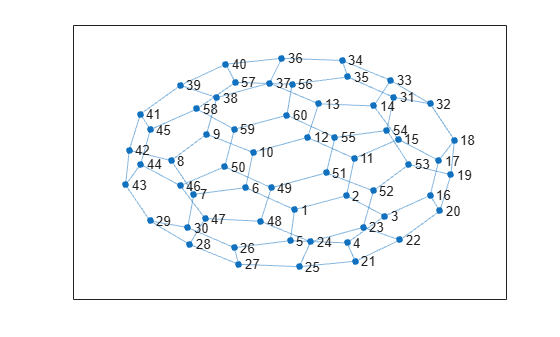```p = GraphPlot with properties: NodeColor: [0 0.4470 0.7410] MarkerSize: 4 Marker: 'o' EdgeColor: [0 0.4470 0.7410] LineWidth: 0.5000 LineStyle: '-' NodeLabel: {1x60 cell} EdgeLabel: {} XData: [1x60 double] YData: [1x60 double] ZData: [1x60 double] Show all properties ```

After you have a handle to the `GraphPlot` object, use dot indexing to access or change the property values. For a complete list of the properties that you can adjust, see GraphPlot Properties.

Change the value of `NodeColor` to `'red'`.

`p.NodeColor = 'red';`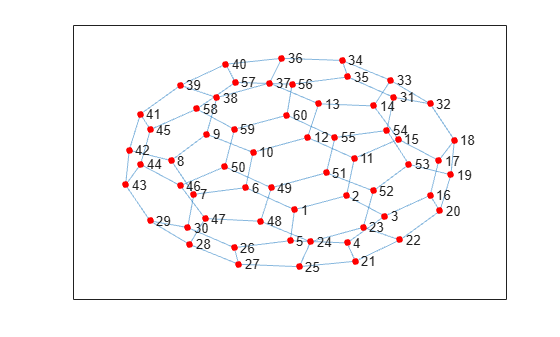Determine the line width of the edges.

`p.LineWidth`
```ans = 0.5000 ```

### Create and Plot Graph

Create and plot a graph representing an L-shaped membrane constructed from a square grid with a side of 12 nodes. Specify an output argument with `plot` to return a handle to the `GraphPlot` object.

```n = 12; A = delsq(numgrid('L',n)); G = graph(A,'omitselfloops')```
```G = graph with properties: Edges: [130x2 table] Nodes: [75x0 table] ```
`p = plot(G)````p = GraphPlot with properties: NodeColor: [0 0.4470 0.7410] MarkerSize: 4 Marker: 'o' EdgeColor: [0 0.4470 0.7410] LineWidth: 0.5000 LineStyle: '-' NodeLabel: {1x75 cell} EdgeLabel: {} XData: [1x75 double] YData: [1x75 double] ZData: [1x75 double] Show all properties ```

### Change Graph Node Layout

Use the `layout` function to change the layout of the graph nodes in the plot. The different layout options automatically compute node coordinates for the plot. Alternatively, you can specify your own node coordinates with the `XData`, `YData`, and `ZData` properties of the `GraphPlot` object.

Instead of using the default 2-D layout method, use `layout` to specify the `'force3'` layout, which is a 3-D force directed layout.

```layout(p,'force3') view(3)```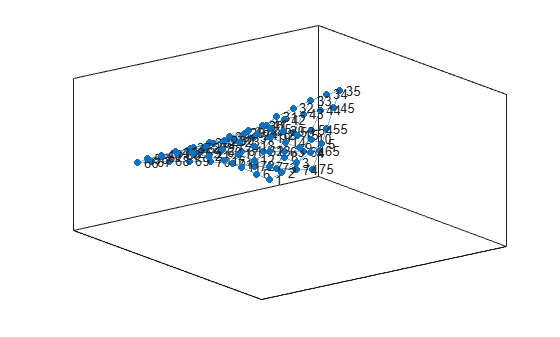### Proportional Node Coloring

Color the graph nodes based on their degree. In this graph, all of the interior nodes have the same maximum degree of 4, nodes along the boundary of the graph have a degree of 3, and the corner nodes have the smallest degree of 2. Store this node coloring data as the variable `NodeColors` in `G.Nodes`.

```G.Nodes.NodeColors = degree(G); p.NodeCData = G.Nodes.NodeColors; colorbar```### Edge Line Width by Weight

Add some random integer weights to the graph edges, and then plot the edges such that their line width is proportional to their weight. Since an edge line width approximately greater than 7 starts to become cumbersome, scale the line widths such that the edge with the greatest weight has a line width of 7. Store this edge width data as the variable `LWidths` in `G.Edges`.

```G.Edges.Weight = randi([10 250],130,1); G.Edges.LWidths = 7*G.Edges.Weight/max(G.Edges.Weight); p.LineWidth = G.Edges.LWidths;```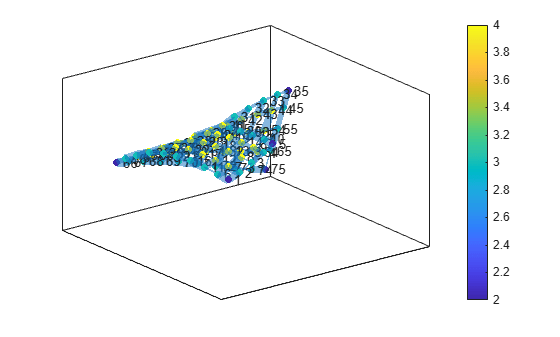### Extract Subgraph

Extract and plot the top right corner of `G` as a subgraph, to make it easier to read the details on the graph. The new graph, `H`, inherits the `NodeColors` and `LWidths` variables from `G`, so that recreating the previous plot customizations is straightforward. However, the nodes in `H` are renumbered to account for the new number of nodes in the graph.

```H = subgraph(G,[1:31 36:41]); p1 = plot(H,'NodeCData',H.Nodes.NodeColors,'LineWidth',H.Edges.LWidths); colorbar```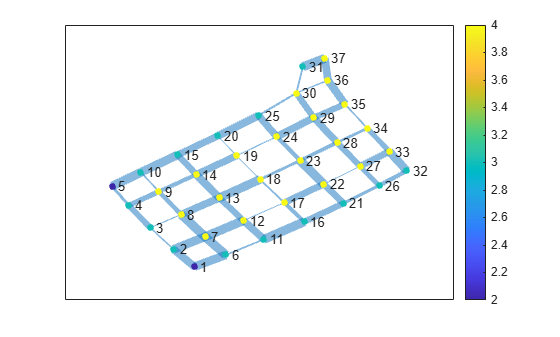### Label Nodes and Edges

Use `labeledge` to label the edges whose width is larger than `6` with the label, `'Large'`. The `labelnode` function works in a similar manner for labeling nodes.

`labeledge(p1,find(H.Edges.LWidths > 6),'Large')`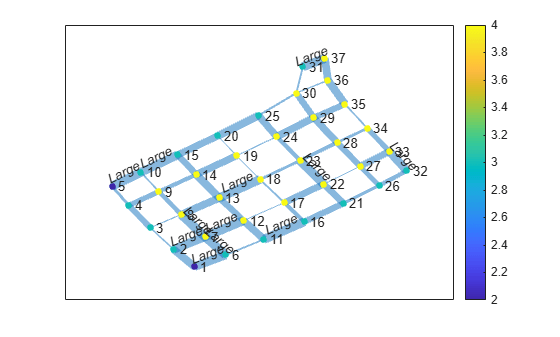### Highlight Shortest Path

Find the shortest path between node 11 and node 37 in the subgraph, `H`. Highlight the edges along this path in red, and increase the size of the end nodes on the path.

`path = shortestpath(H,11,37)`
```path = 1×10 11 12 17 18 19 24 25 30 36 37 ```
```highlight(p1,[11 37]) highlight(p1,path,'EdgeColor','r')```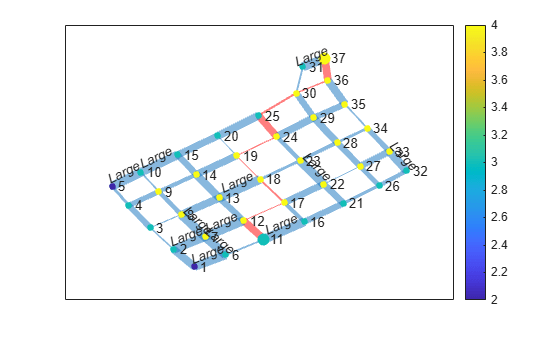Remove the node labels and colorbar, and make all of the nodes black.

```p1.NodeLabel = {}; colorbar off p1.NodeColor = 'black';```Find a different shortest path that ignores the edge weights. Highlight this path in green.

`path2 = shortestpath(H,11,37,'Method','unweighted')`
```path2 = 1×10 11 12 13 14 15 20 25 30 31 37 ```
`highlight(p1,path2,'EdgeColor','g')`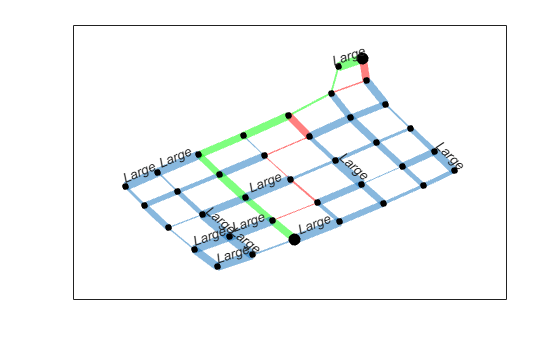### Plotting Large Graphs

It is common to create graphs that have hundreds of thousands, or even millions, of nodes and/or edges. For this reason, `plot` treats large graphs slightly differently to maintain readability and performance. The `plot` function makes these adjustments when working with graphs that have more than 100 nodes:

1. The default graph layout method is always `'subspace'`.

2. The nodes are no longer labeled automatically.

3. The `MarkerSize` property is set to `2`. (Smaller graphs have a marker size of `4`).

4. The `ArrowSize` property of directed graphs is set to `4`. (Smaller directed graphs use an arrow size of `7`).

Download ebook